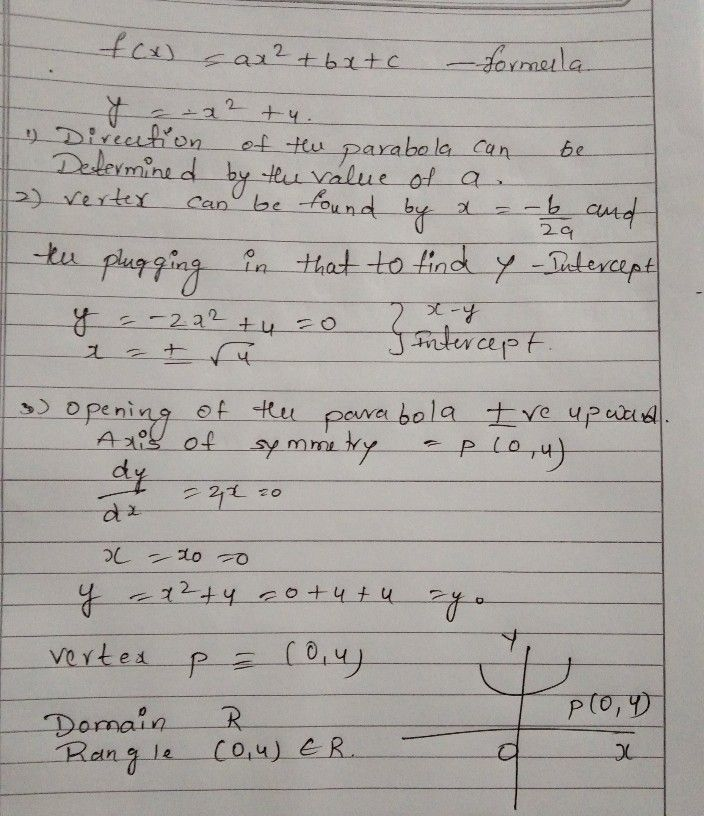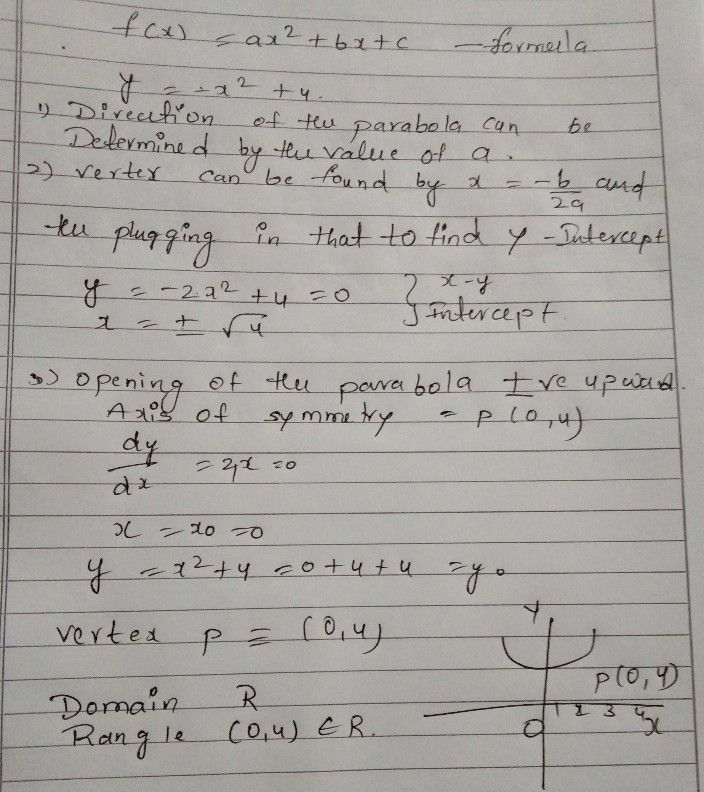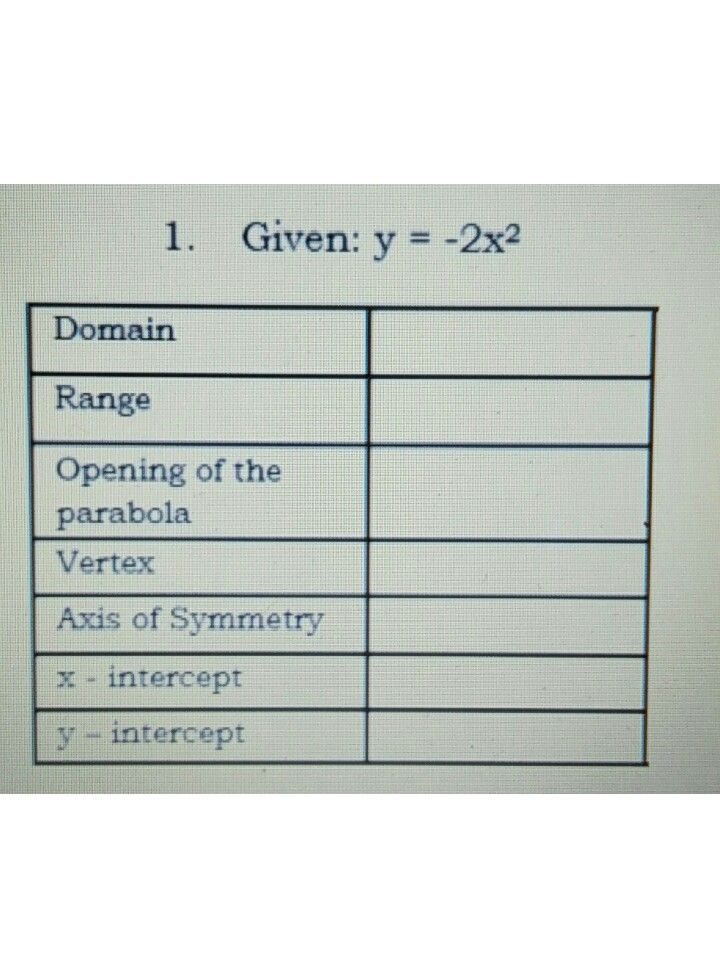Symbol
Problem$2$ Given: $y=-x^{2+4}$ Domain Range Opening of the parabola Vertex Axis of Symmetry $x=1$ intercept $y-intcttep$
7th-9th grade
Geometry
Search count: 108
Question content
please complete the tables below and graph on the same coordinate plane.
SolutionQanda teacher - sathishStudentthankyou so much for your answer sir.